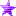# Outerra forum

• July 12, 2020, 09:49:24 am• Welcome, Guest

Pages: 

###AuthorTopic: slow scenery loading in new version  (Read 1018 times)

#### fly77

• Hero Member
•• Posts: 934« on: March 26, 2019, 02:59:09 am »

Code: [Select]
`time-of-day = 61987251.58637,day-of-year = 93,daytime-multiplier = 1.0,terrain-rpf = 6,tree-quality = 0.2,prepare-levels-rel = 0,max-level-terrain = 11,limit-wavelet = 128,limit-lastelev = 96,limit-fractal = 560,limit-nonbaked = 128,limit-treetiles = 96,limit-grasstiles = 60,trees = true,tree-bb-dist = 0.0,max-obj-dist = 0.0,obj-count-threshold = 0,planet = "earth7",moon-scale = 4.0,max-quality-vres = 480,object-level-shift = 0,texture-mod = "",ufo-speed-mul = 3.0,ufo-speed-exp = 1.75,ufo-speed-max = 200000.0,ufo-speed-boost = 5.0,ufo-hud-config = { speed = "km/h", dist = "m", alt = "m", imperial_aircraft_units = true},grass = true,grass-distance = 45.0,grass-half-Q = true,grass-lod1 = 10.0,clouds = true,last-import-path = "",last-export-path = "",terrain-normal-maps = true,first-run = false,forest_params = { render_distcoef = 1, shadow_range = 0.25, shading = true},env_water = false,locklevel = 1,lockbelow = 25.0,data-mask = 232,data-path = "data",cfg-version = 10447`

Code: [Select]
`num_jobproc_threads = 1,renderer_config = { screen_width = 2560, screen_height = 1080, fullscreen = true, swap_interval = 0, msaa = 1, debug_opengl = false, debug_async = true, refresh_rate = 60},videos_dir = "I:/outerra-videos\videos",screenshots_dir = "C:/Users/novelchip/Outerra\screenshots",terrain_shadows = true,tree_shadows = true,wnd_x_pos = 0,wnd_y_pos = 0,wnd_hide_titlebar = true,mouse_exclusive = false,sim_mode = false,fov = 63.799999,fov_v_separate = 0.0,proj = 0,enable_hid_logging = false,enable_jsbsim_logging = false,mouse_sensitivity = 1.0,joy_sensitivity = 1.0,mouse_invert = false,flightmode_invert = true,pause_in_sandbox = true,debug_keys = false,cfg-version = 10447,bloom_power = 0.03,bloom_threshold = 1.0,min_obj_size = 5.0,sound = { global = 1.0, ambient = 1.0, vehicle = 0.5, effect = 0.09, music = 1.0, hrtf = true},use_hmd = false,video = 1,ssao_quality = 2,shadow_quality = 2,stereo = { eye_separation = 0.064, convergence = 0.1, reduce_grass = false, force_hrtf = true, stereo_type = 0, swap_sides = false},controller = { pan_min_angle = { x = -1.570796, y = -0.785398 }, pan_max_angle = { x = 1.570796, y = 0.785398 }, panning_speed = { x = 0.01, y = 0.01 }, view_min_angle = { x = -1.570796, y = -0.785398 }, view_max_angle = { x = 1.570796, y = 0.785398 }, view_delay = 0.1, fm_roll_coef = 0.4, fm_turn_coef = 1.25, fm_turn_delay = 4.0, fm_filter_delay = 0.5, um_filter_delay = 0.02, hmd_turn_coef = 1.0, hmd_pitch_coef = 0.2, vr_ufo_pitch = false, vr_mouse_pitch = false, vr_ufo_ref = 2, vr_fps_ref = 3},perf_report = { enabled = false, sync = false, ui = 5.0, scene = 5.0, world = 5.0, ext = 5.0, rungc = 0},pkg_limits = { max_meshes = 4},disable_log_popup = false,img_cache_slice_size = 2048,img_cache_num_slices = 2,loglevel = "info"`
« Last Edit: March 26, 2019, 03:23:00 am by fly77 »Logged

#### cameni

• Brano Kemen
• Hero Member
•• Posts: 6668
•• Pegs is clever, but tae hain’t a touch sentimental« Reply #1 on: March 26, 2019, 09:12:08 am »

It's a known issue as we are transitioning to new pipeline and x64 builds, and some code changes merged into this version are having unexpected side effects.Logged

#### fly77

• Hero Member
•• Posts: 934Logged